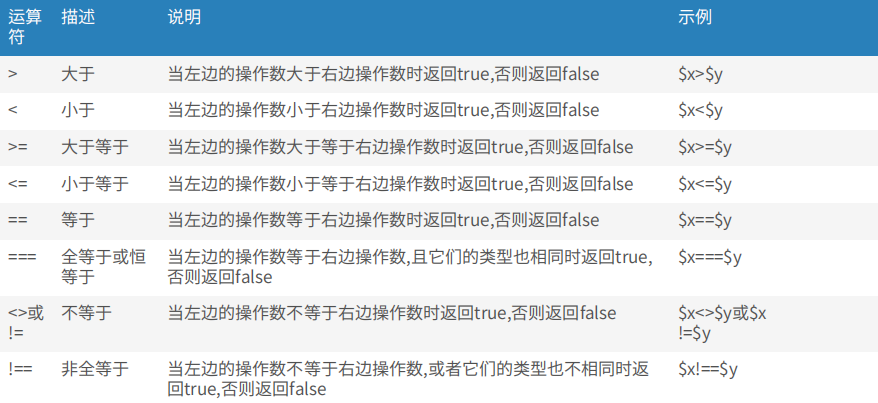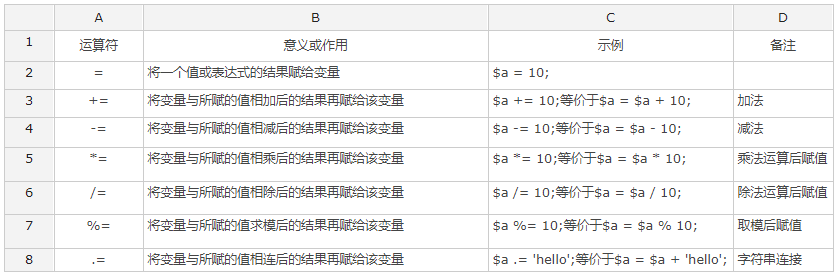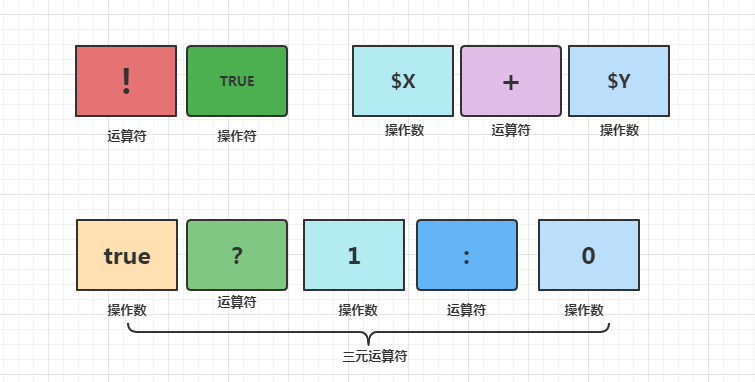# PHP 8: PHP的运算符

+关注继续查看

PHP运算符有很多种，看样子要比C/C++,C#等语言多多了。分别是：

• 算术运算符
• 赋值运算符
• 位运算符
• 比较运算符
• 错误控制运算符
• 执行运算符
• 递增／递减运算符
• 逻辑运算符
• 字符串运算符
• 数组运算符
• 类型运算符

 例子 名称 结果 -$a 取反$a 的负值。 $a +$b 加法 $a 和$b 的和。 $a -$b 减法 $a 和$b 的差。 $a *$b 乘法 $a 和$b 的积。 $a /$b 除法 $a 除以$b 的商。 $a %$b 取模 $a 除以$b 的余数。

 例子 名称 结果 $a &$b And（按位与） 将把 $a 和$b 中都为 1 的位设为 1。 $a |$b Or（按位或） 将把 $a 或者$b 中为 1 的位设为 1。 $a ^$b Xor（按位异或） 将把 $a 和$b 中不同的位设为 1。 ~ $a Not（按位非） 将$a 中为 0 的位设为 1，反之亦然。 $a <<$b Shift left（左移） 将 $a 中的位向左移动$b 次（每一次移动都表示“乘以 2”）。 $a >>$b Shift right（右移） 将 $a 中的位向右移动$b 次（每一次移动都表示“除以 2”）。

 例子 名称 结果 $a ==$b 等于 TRUE，如果 $a 等于$b。 $a ===$b 全等 TRUE，如果 $a 等于$b，并且它们的类型也相同。（PHP 4 引进） $a !=$b 不等 TRUE，如果 $a 不等于$b。 $a <>$b 不等 TRUE，如果 $a 不等于$b。 $a !==$b 非全等 TRUE，如果 $a 不等于$b，或者它们的类型不同。（PHP 4 引进） $a <$b 小与 TRUE，如果 $a 严格小于$b。 $a >$b 大于 TRUE，如果 $a 严格$b。 $a <=$b 小于等于 TRUE，如果 $a 小于或者等于$b。 $a >=$b 大于等于 TRUE，如果 $a 大于或者等于$b。

 例子 名称 效果 ++$a 前加$a 的值加一，然后返回 $a。$a++ 后加 返回 $a，然后将$a 的值加一。 --$a 前减$a 的值减一， 然后返回 $a。$a-- 后减 返回 $a，然后将$a 的值减一。

 例子 名称 结果 $a and$b And（逻辑与） TRUE，如果 $a 与$b 都为 TRUE。 $a or$b Or（逻辑或） TRUE，如果 $a 或$b 任一为 TRUE。 $a xor$b Xor（逻辑异或） TRUE，如果 $a 或$b 任一为 TRUE，但不同时是。 ! $a Not（逻辑非） TRUE，如果$a 不为 TRUE。 $a &&$b And（逻辑与） TRUE，如果 $a 与$b 都为 TRUE。 $a ||$b Or（逻辑或） TRUE，如果 $a 或$b 任一为 TRUE。

 例子 名称 结果 $a +$b 联合 $a 和$b 的联合。 $a ==$b 相等 如果 $a 和$b 具有相同的键／值对则为 TRUE。 $a ===$b 全等 如果 $a 和$b 具有相同的键／值对并且顺序和类型都相同则为 TRUE。 $a !=$b 不等 如果 $a 不等于$b 则为 TRUE。 $a <>$b 不等 如果 $a 不等于$b 则为 TRUE。 $a !==$b 不全等 如果 $a 不全等于$b 则为 TRUE。

• 首先看看位运算里的左移(<<)或右移(>>)，其实没有什么不同，只是符号不一样而已。
• 看看比较运算法里的“＝＝”，“＝＝＝”,这两个比较有意思，它们有什么区别呢？
看看一下代码：
1 <?php
2 var_dump(0 == "a"); // 0 == 0 -> true
3 var_dump("1" == "01"); // 1 == 1 -> true
4
5 switch ("a") {
6 case 0:
7    echo "0";
8    break;
9 case "a": // never reached because "a" is already matched with 0
10    echo "a";
11    break;
12 }
看第2行，为什么0会等于“a”呢？奇怪了。之所以会相等，是因为在PHP里，如果整型和字符串比较时，字符串会自动的转化为数值。这里就会有一个问题：字符串转化为数值的原则是什么？原则是

1）如果包括“.”，“e”或“E”其中任何一个字符的话，字符串被当作 float 来求值。否则就被当作整数。

2）该值由字符串最前面的部分决定。如果字符串以合法的数字数据开始，就用该数字作为其值，否则其值为 0（零）。合法数字数据由可选的正负号开始，后面跟着一个或多个数字（可选地包括十进制分数），后面跟着可选的指数。指数是一个“e”或者“E”后面跟着一个或多个数字。例如：

1 <?php
2 $var = 1 + "10.5"; 3 echo "1 + \"10.5\"=".$var."; type:".gettype($var)."<Br>"; 4$var = 1 + "-1.3e3";
5 echo "1 + \"-1.3e3\"=".$var."; type:".gettype($var)."<Br>";
6 $var = 1 + "bob-1.3e3"; 7 echo "1 + \"bob-1.3e3\"=".$var."; type:".gettype($var)."<Br>"; 8$var = 1 + "bob3";
9 echo "1 + \"bob3\"=".$var."; type:".gettype($var)."<Br>";
10 $var = 1 + "10 beatiful birds"; 11 echo "1 + \"10 beatiful birds\"=".$var."; type:".gettype($var)."<Br>"; 12$var= 4 + "10.2 Little Apple"
13 echo "4 + \"10.2 Little Apple\"=".$var."; type:".gettype($var)."<Br>";
14 $var = "10.0 pigs " + 1; 15 echo "\"10.0 pigs \" + 1=".$var."; type:".gettype($var)."<Br>"; 16$var = "10.0 pigs " + 1.0;
17 echo  "\"10.0 pigs \" + 1.0=".$var."; type:".gettype($var)."<Br>";
18 ?>
19

1 + "10.5"=11.5; type:double
1 + "-1.3e3"=-1299; type:double
1 + "bob-1.3e3"=1; type:integer
1 + "bob3"=1; type:integer
1 + "10 beatiful birds"=11; type:integer
4 + "10.2 Little Apple"=14.2; type:double
"10.0 pigs " + 1=11; type:double
"10.0 pigs " + 1.0=11; type:double

1 <?php
2  var_dump(0 === "a"); // 0 === 0 -> false
3  echo '<br>';
4  var_dump(0 === "00"); // 0 === "00" -> false
5  echo '<br>';
6  var_dump(0 === 0); // 0 === 0 -> true
7  echo '<br>';
8 ?>

bool(false)
bool(
false)
bool(
true

3）看看逻辑运算，“或”和“与”居然有2种写法，如果你使用C/C++,C#,Java等语言的话，就用“&&"或”||"吧。如果你是VB的话，就用and，和or。当然你也可以2种都用。它们有什么区别呢？优先级的不同而已。”&&“与"||"比”and“和”or“优先级要高。
4）.PHP多了一个数组运算符。有了上面的解释，相信它们不是很难了。

• 赋值运算符
和C#语言一样，也是利用”＝“，也是利用二元符号赋值。例如：
<?php

$a='hello'//$a是hello。
$a+=1; //$a是1，二元赋值符
$b='world';$c='hello';

$c.=$b;//$c是"hello world" ?> • 错误执行运算符 PHP 支持一个错误控制运算符：@。当将其放置在一个 PHP 表达式之前，该表达式可能产生的任何错误信息都被忽略掉。 如果激活了 track_errors 特性，表达式所产生的任何错误信息都被存放在变量$php_errormsg 中。此变量在每次出错时都会被覆盖，所以如果想用它的话就要尽早检查。例如：

<?php
/* Intentional file error */
$my_file = @file ('non_existent_file') or die ("Failed opening file: error was '$php_errormsg'");

// this works for any expression, not just functions:
$value = @$cache[$key]; // will not issue a notice if the index$key doesn't exist.

?>

• 执行运算符
PHP 支持一个执行运算符：反引号（）。注意这不是单引号！PHP 将尝试将反引号中的内容作为外壳命令来执行，并将其输出信息返回（例如，可以赋给一个变量而不是简单地丢弃到标准输出）。使用反引号运算符“”的效果与函数 shell_exec() 相同。反引号位于键盘的”~“键那里。例如：
1 <?php
2  $out=dir c:`; 3 echo '<pre>'.$out.'</pre>';
4 ?>

Volume in drive C has no label.
Volume Serial
Number is A019-7D77

Directory of C
:\

05/13/2006 07:07 PM 220 cmd.txt
02/13/2006 11:40 PM WINDOWS
02/13/2006 11:47 PM Documents and Settings
02/13/2006 11:57 PM Program Files
06
/24/2006 11:01 PM 482,933 nfzmLog.log
05
/11/2006 10:28 PM  Inetpub
05
/13/2006 07:07 PM 4 response.txt
07/29/2006 05:56 PM 0 Rec.pcm
06/24/2006 09:05 PM nf
07/29/2006 05:56 PM 0 WriteAudrv.wav
07/04/2006 08:52 PM dwnSetup
07/08/2006 09:28 AM TEMP
02/14/2006 12:15 AM NVIDIA
5 File(s) 483,157 bytes 10 Dir(s) 2,116,558,848 bytes free

• # 递增／递减运算符

PHP 支持 C 风格的前／后递增与递减运算符。

注: 递增／递减运算符不影响布尔值。递减 NULL 值也没有效果，但是递增 NULL 的结果是 1。

递增／递减运算符

例子 名称 效果
++$a 前加$a 的值加一，然后返回 $a。$a++ 后加 返回 $a，然后将$a 的值加一。
--$a 前减$a 的值减一， 然后返回 $a。$a-- 后减 返回 $a，然后将$a 的值减
• # 类型运算符

PHP 有一个类型运算符：instanceof。instanceof 用来测定一个给定的对象是否来自指定的对象类。

instanceof 运算符是 PHP 5 引进的。在此之前用 is_a()，但是 is_a() 已经过时了，最好用 instanceof。

C#里是is，而Java是instanceof，和PHP一样。例如：
1 <?php
2 class A { }
3 class B { }
4
5 $thing = new A; 6 7 if ($thing instanceof A) {
8     echo 'A';
9 }
10 if ($thing instanceof B) { 11 echo 'B'; 12 } 13 ?> • 字符运算符 有两个字符串运算符。第一个是连接运算符（“.”），它返回其左右参数连接后的字符串。第二个是连接赋值运算符（“.=”），它将右边参数附加到左边的参数后。这些在前面已经见到，就不举例了。 版权声明：本文内容由阿里云实名注册用户自发贡献，版权归原作者所有，阿里云开发者社区不拥有其著作权，亦不承担相应法律责任。具体规则请查看《阿里云开发者社区用户服务协议》和《阿里云开发者社区知识产权保护指引》。如果您发现本社区中有涉嫌抄袭的内容，填写侵权投诉表单进行举报，一经查实，本社区将立刻删除涉嫌侵权内容。作者高分内容 更多 相关文章【web 开发基础】PHP 快速入门（7）-PHP 运算符之比较运算符详解 比较运算符也称关系运算符，又称条件运算符，也是一种常用的二元运算符，用于对运算符两边的操作数或者表达式进行比较。比较运算符的结果只能是布尔值。如果比较的关系为真，则结果为TRUE；否则结果为FALSE。 程序的世界很奇妙，但是程序员的奇妙你想不到。比如下面的故事就很奇妙（哈哈哈~）： 0 0【web 开发基础】PHP 快速入门（6）-PHP 运算符之赋值运算符详解 上面是 PHP 开发基础快速入门系列的文章,通过前面几篇文章的介绍我们或多或少对PHP的开发有所了解。我们了解了PHP相关的基本概念以及简单的用法，正所谓万事开头难，这已经到了第6篇，说明我们已经度过了最难的时候，开弓没有回头箭，接着继续学习PHP开发的相关内容。本文接着介绍PHP运算符相关的知识--赋值运算符。了解赋值运算符我们依然不落俗套，首先来了解它是什么，再了解它怎么用。 0 0【web开发基础】PHP快速入门（5）-PHP运算符之算术运算符和字符串运算符详解 运算符用于执行程序代码运算，会针对一个以上操作数项目来进行运算。例如:2+3,其操作数是2和3，而运算符则是"+"。如在vb2005中运算符大致可以分为5种类型:算术运算符、连接运算符、关系运算符、赋值运算符和逻辑运算符；PHP也同样包含这些运算符。 运算符和变量是所有计算机编程语言中的不可或缺的一部分，是一个命令解释器对一个或多个操作数（变量或数值）执行某种运算的符号，也称为操作符 0 0快速入门PHP第一天，常用标识符，运算符，循环语句❤❤ 快速入门PHP第一天，常用标识符，运算符，循环语句❤❤ 0 0php运算符 987 0php运算符优先级 584 0PHP ! 非运算符 与 if 判断深入研究 610 0php学习之二运算符 定义常量函数 define(string,value,F|T) string 常量名字(字符串,可以不用$)   value 常量的值     F|T   大小写是否敏感,默认false 敏感  一般不动 系统常量 （1）__FILE__ :php程序文件名。
532 0

PHP进阶教程 - 由浅入深掌握面向对象开发 - 第一阶段20332

PHP进阶教程 - 由浅入深掌握面向对象开发 - 第二阶段13733

PHP进阶教程 - 由浅入深掌握面向对象开发 - 第三阶段20831

PHP完全自学手册文档教程940588

PHP安全开发_从白帽角度做安全

PHP在机器学习上的应用及云深度学习平台的架构设计与实现

PHP与APM_技术内幕和最佳实践